# Beginner’s Guide to Implement Text Classification (Sentiment Analysis) Project using NLP

Sentiment analysis is a popular NLP (Natural Language Processing) operation with many applications, from analyzing customer reviews to gauging public sentiment on social media.

Text classification, also known as text tagging or text categorization, is the process of categorizing text into organized groups.

Using NLP, text classifiers can automatically analyze text and assign pre-defined tags or categories based on its content.

## Goal of this Machine Learning Project

Before we start, let me define the explicit goal of this tutorial. This is a hands-on sentiment analysis project.

For sentiment analysis, we will implement Multiclass Sentiment Analysis (Categorizes sentiment into multiple classes, such as positive, negative, and neutral.) on the Dataset of Starbucks reviews.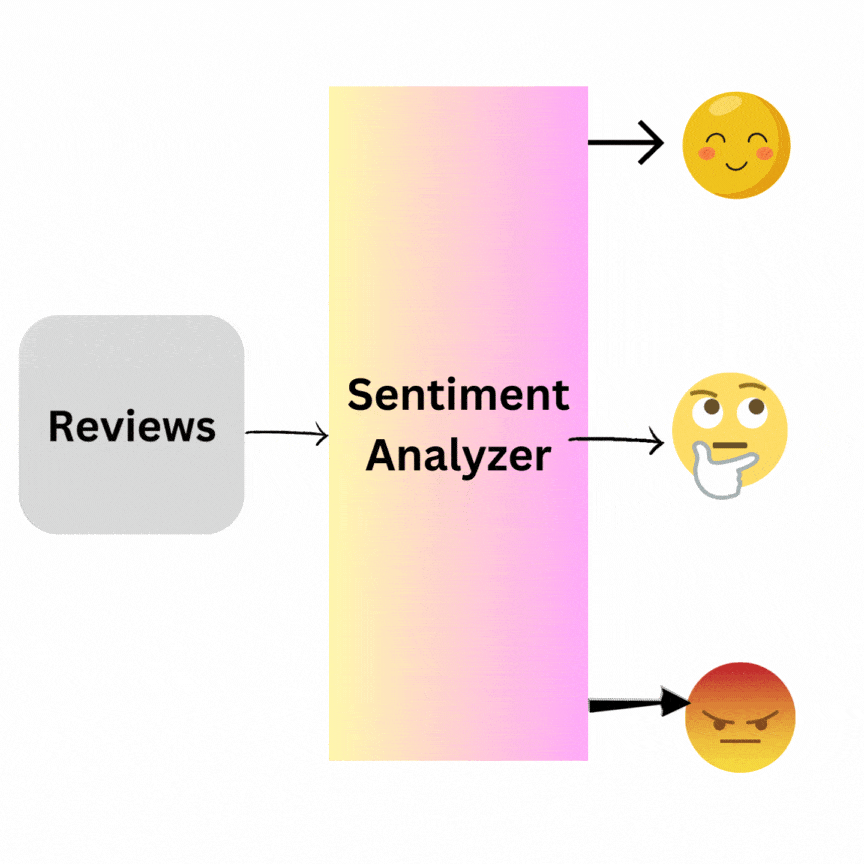## Install Libraries

Before implementing the Text classification program, you must install the libraries below.

1. Pandas: You can install it using this command: pip install pandas
2. nltk: You can install it using this command: pip install nltk
3. stopwords: You can install it using this command: python3 -m nltk.downloader stopwords
4. scikit-learn: You can install it using this command: pip install -U scikit-learn

## Flow Diagram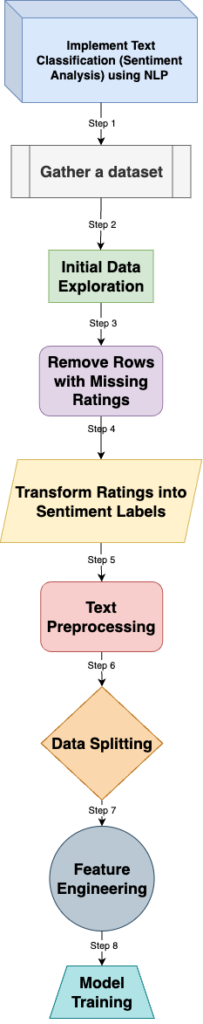## Step 1: Gather a dataset

For this tutorial, we will use Kaggle’s Starbucks Reviews Dataset. I downloaded the Dataset in my project as reviews_data.csv. I highly recommend checking out the Kaggle website for different datasets to train your model.

Let’s load and examine the Dataset.

``````import pandas as pd

# Display the first few rows of the dataset
``````

Output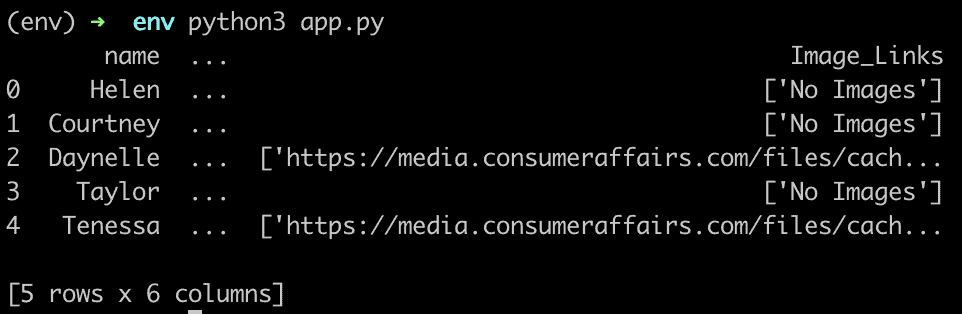The main columns of interest for our sentiment analysis project are the Review (feature) and Rating (target) columns.

## Step 2: Initial Data Exploration

Before going further, we must analyze the data and check for the missing values. If we train the model using missing values, then the model won’t train correctly. The process is called data cleaning.

The next step would be to get a summary of the rating distribution and examine the length of reviews to see if we have very short or very long reviews that might need special attention.

``````# Checking for missing values
missing_values = reviews_df.isnull().sum()

# Summary of the distribution of ratings
rating_distribution = reviews_df['Rating'].value_counts(normalize=True) * 100

# Examine the length of reviews
reviews_df['Review_Length'] = reviews_df['Review'].apply(lambda x: len(str(x)
.split()))
review_length_stats = reviews_df['Review_Length'].describe()

print(missing_values, rating_distribution, review_length_stats)``````

Output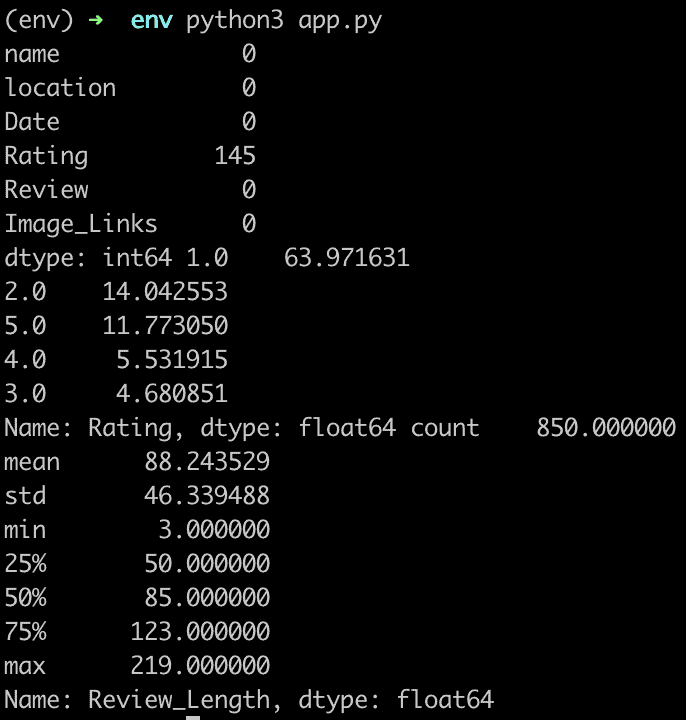Here’s what we have observed from our initial exploration:

1. Missing Values:
• The Rating column has 145 missing values. We have to decide how to handle these. We could remove these entries or impute them based on specific criteria.
• Other columns don’t have missing values relevant to our sentiment analysis task.
2. Rating Distribution:
• The majority (about 64%) of reviews have a rating of 1.0.
• About 14% have a rating of 2.0, and about 12% have a rating of 5.0.
• Ratings of 4.0 and 3.0 are less frequent, with approximately 5.5% and 4.7% respectively.
• This indicates an imbalanced dataset, which we must consider during modeling.
3. Review Length:
• The average review length is about 88 words.
• The shortest review is three words, while the longest is 219 words.
• The median length is 85 words.
• Most reviews (75th percentile) are under 123 words in length.

## Step 3: Remove Rows with Missing Ratings

Before we proceed further, let’s handle the missing values in the Rating column.

Since it’s our target variable, dropping rows with missing ratings is the most straightforward approach.

``````# Removing rows with missing ratings
reviews_df_cleaned = reviews_df.dropna(subset=['Rating']).copy()

# Checking the shape of the cleaned dataset
new_shape = reviews_df_cleaned.shape

print(new_shape)
``````

Output

``````(705, 7)
``````

After dropping the missing values, you can see that the cleaned Dataset now contains 705 entries.

## Step 4: Transform Ratings into Sentiment Labels

For sentiment analysis, we often categorize sentiments into three classes

1. “Positive”: Ratings 4.0 and 5.0
2. Neutral”: Rating 3.0
3. “Negative”: Ratings 1.0 and 2.0

Let’s transform the Rating column into these sentiment labels.

``````# Transforming ratings into sentiment labels
def rating_to_sentiment(rating):
if rating in [1.0, 2.0]:
return 'Negative'
elif rating == 3.0:
return 'Neutral'
else:
return 'Positive'

reviews_df_cleaned['Sentiment'] = reviews_df_cleaned['Rating'].apply(rating_to_sentiment)

# Checking the distribution of the new Sentiment column
sentiment_distribution = reviews_df_cleaned['Sentiment'].value_counts(normalize=True) * 100

print(sentiment_distribution)
``````

Output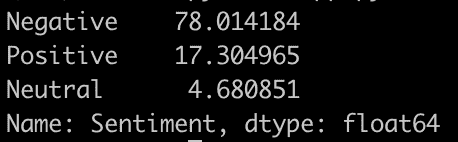The sentiment distribution in our cleaned Dataset is as follows:

1. Negative: Approximately 78%
2. Positive: Approximately 17.3%
3. Neutral: Approximately 4.7%

This confirms the earlier observation of an imbalanced dataset with many negative reviews.

## Step 5: Text Preprocessing

To prepare the reviews for modeling, we’ll perform the following text preprocessing steps:

1. Convert text to lowercase for uniformity.
2. Tokenize the reviews (split them into words).
3. Remove stop words (common words that might not add significant meaning in sentiment analysis).
4. Apply stemming (reduce words to their root/base form).

We will start by defining functions for each preprocessing step and then apply them to the Review column.

``````from nltk.tokenize import word_tokenize
from nltk.corpus import stopwords
from nltk.stem import PorterStemmer

# Initialize stemmer and set of stopwords
stemmer = PorterStemmer()
stop_words = set(stopwords.words('english'))

def preprocess_review(text):
# Convert to lowercase
text = text.lower()

# Tokenize
tokens = word_tokenize(text)

# Remove stopwords and apply stemming
tokens = [stemmer.stem(token) for token in tokens if token.isalpha() and token not in stop_words]

return ' '.join(tokens)

# Apply preprocessing to the Review column
reviews_df_cleaned['Processed_Review'] = reviews_df_cleaned['Review'].apply(preprocess_review)

# Display some of the processed reviews

Output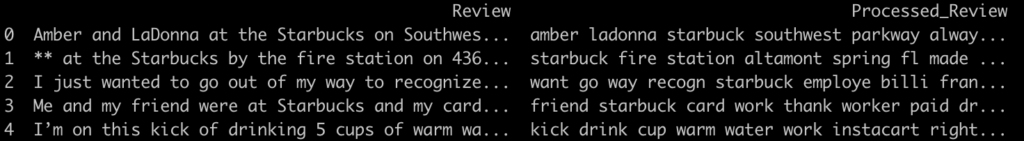The preprocessing has been successfully applied to the reviews:

1. We have converted the text to lowercase.
2. Tokenized the reviews into words.
3. Removed common stopwords.
4. Applied stemming to reduce words to their base form.

You can see that now the Processed_Review column contains the cleaned and processed versions of the reviews.

## Step 6: Data Splitting

Before modeling, we need to split the data into training and test sets. This allows us to train our model on one subset and test its performance on another unseen subset.

We will split the data into a training set and a test set. A common practice is to use 80% of the data for training and 20% for testing.

``````from sklearn.model_selection import train_test_split

# Features and target variable
X = reviews_df_cleaned['Processed_Review']
y = reviews_df_cleaned['Sentiment']

# Splitting the data into training and test sets (80% - 20%)
X_train, X_test, y_train, y_test = train_test_split(X, y, test_size=0.2,
random_state=42, stratify=y)
print(X_train.shape, X_test.shape)``````

Output

``````(564,) (141,)
``````

The data has been successfully split:

1. Training set: 564 samples
2. Test set: 141 samples

## Step 7: Feature Engineering

Before training a machine learning model, we must convert our processed reviews into a format the model can understand.

One standard method is the Term Frequency-Inverse Document Frequency (TF-IDF) technique, which transforms the text data into numerical vectors while considering the importance of each word in the Dataset.

We will use the TfidfVectorizer from scikit-learn to convert our processed reviews into numerical vectors. This vectorizer will be fit on the training data and then used to transform the training and test data.

``````from sklearn.feature_extraction.text import TfidfVectorizer

# Initialize the TF-IDF vectorizer
tfidf_vectorizer = TfidfVectorizer(max_features=5000, ngram_range=(1,2))

# Fit and transform the training data
X_train_tfidf = tfidf_vectorizer.fit_transform(X_train)

# Transform the test data
X_test_tfidf = tfidf_vectorizer.transform(X_test)

print(X_train_tfidf.shape, X_test_tfidf.shape)``````

Output

``(564, 5000) (141, 5000)``

The training and test sets have been transformed into numerical vectors with 5,000 features (or terms) each.

## Step 8: Model Training

We will start with a simple yet effective model: the Logistic Regression classifier for our sentiment analysis task.

The Logistic Regression model works well for binary and multi-class classification problems, especially as a starting point.

Let’s start training a Logistic Regression Classifier.

We will initialize and train a Logistic Regression classifier using the training data.

After training, we will evaluate its performance on the test data to understand its accuracy.

``````from sklearn.linear_model import LogisticRegression
from sklearn.metrics import accuracy_score, classification_report

# Initialize the Logistic Regression classifier
logreg = LogisticRegression(max_iter=1000, random_state=42)

# Train the model
logreg.fit(X_train_tfidf, y_train)

# Predict on the test set
y_pred = logreg.predict(X_test_tfidf)

# Evaluate the model's performance
accuracy = accuracy_score(y_test, y_pred)
classification_rep = classification_report(y_test, y_pred)

print(accuracy, classification_rep)``````

Output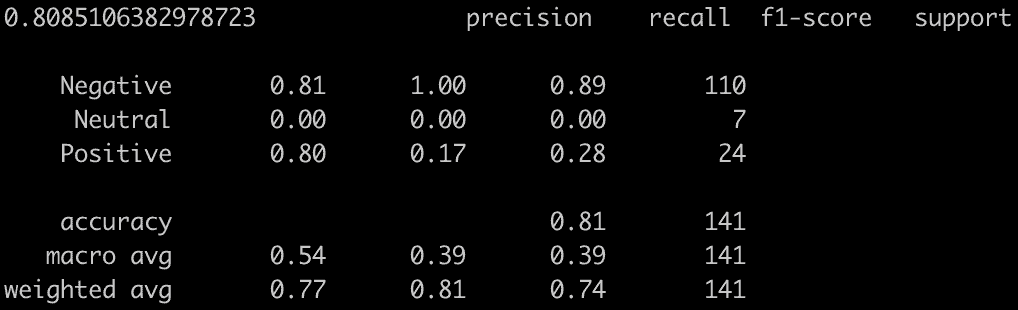The Logistic Regression classifier achieved an accuracy of approximately 80.85%.

• Negative reviews:
• Precision: 0.81
• Recall: 1.00
• F1-Score: 0.89
• Neutral reviews:
• Precision: 0.00
• Recall: 0.00
• F1-Score: 0.00
• Positive reviews:
• Precision: 0.80
• Recall: 0.17
• F1-Score: 0.28

From the results, it’s clear that the model performs well on negative reviews but struggles with neutral and positive reviews. This is expected due to the imbalanced nature of the Dataset, where negative reviews dominate.

## Visualize these metrics for different sentiments using a Bar Chart

A good way to visualize these metrics for different sentiments is using a bar chart.

I will create a grouped bar chart to compare Precision, Recall, and F1-Score across the three sentiment categories: Negative, Neutral, and Positive.

``````import matplotlib.pyplot as plt

# Data for the chart
sentiments = ['Negative', 'Neutral', 'Positive']
precision = [0.81, 0.00, 0.80]
recall = [1.00, 0.00, 0.17]
f1_score = [0.89, 0.00, 0.28]

# Create a bar chart
bar_width = 0.25
r1 = range(len(precision))
r2 = [x + bar_width for x in r1]
r3 = [x + bar_width for x in r2]

plt.figure(figsize=(10, 7))

plt.bar(r1, precision, width=bar_width, label='Precision', color='blue')
plt.bar(r2, recall, width=bar_width, label='Recall', color='green')
plt.bar(r3, f1_score, width=bar_width, label='F1-Score', color='red')

# Adding labels to the chart
plt.xlabel('Sentiment', fontweight='bold')
plt.xticks([r + bar_width for r in range(len(precision))], sentiments)
plt.ylabel('Score', fontweight='bold')
plt.title('Performance Metrics by Sentiment', fontweight='bold')
plt.legend()

# Display the chart
plt.tight_layout()
plt.show()
``````

Output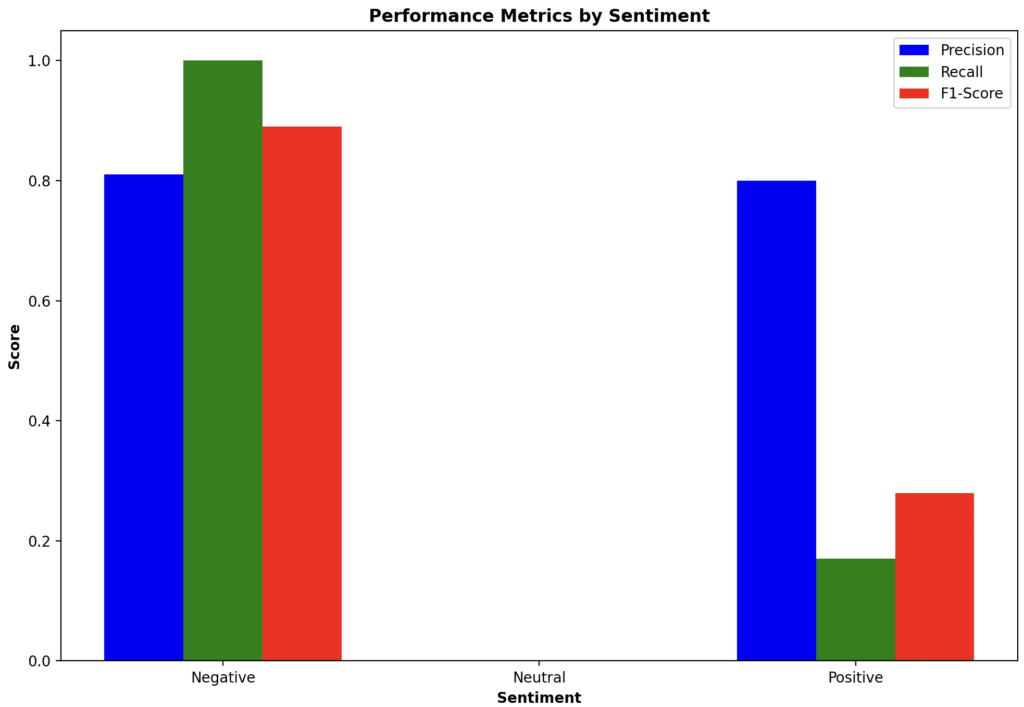You can check out the complete code on Github.

This site uses Akismet to reduce spam. Learn how your comment data is processed.